• Posts tagged "class"

# Blog Archives

## R语言基于R6的面向对象编程

R的极客理想系列文章，涵盖了R的思想，使用，工具，创新等的一系列要点，以我个人的学习和体验去诠释R的强大。

R语言作为统计学一门语言，一直在小众领域闪耀着光芒。直到大数据的爆发，R语言变成了一门炙手可热的数据分析的利器。随着越来越多的工程背景的人的加入，R语言的社区在迅速扩大成长。现在已不仅仅是统计领域，教育，银行，电商，互联网….都在使用R语言。

• 张丹(Conan), 程序员Java,R,PHP,Javascript
• weibo：@Conan_Z
• blog: http://blog.fens.me
• email: bsspirit@gmail.com

http://blog.fens.me/r-class-r6/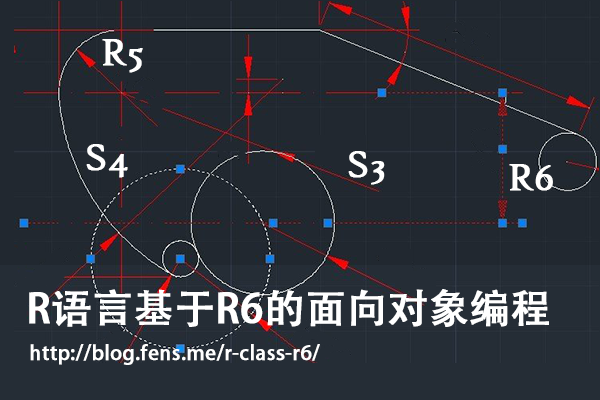R6是什么？听说过S3、S4和RC(R5)的面向对象类型 ，R6难道是一种新的类型吗？

1. 初识R6
2. 创建R6类和实例化对象
3. R6类的主动绑定
4. R6类的继承关系
5. R6类的对象的静态属性
6. R6类的可移植类型
7. R6类的动态绑定
8. R6类的打印函数
9. 实例化对象的存储
10. R6面向对象系统的案例

## 1. 初识R6

R6是一个单独的R包，与我们熟悉的原生的面向对象系统类型S3,S4RC类型不一样。在R语言的面向对象系统中，R6类型与RC类型是比较相似的，但R6并不基于S4的对象系统，因此我们在用R6类型开发R包的时候，不用依赖于methods包，而用RC类型开发R包的时候则必须设置methods包的依赖，在发布gridgame游戏包 文章中，就会出现RC依赖于methods包的使用情况。

R6类型比RC类型更符合其他编程对于面向对象的设置，支持类的公有成员和私有成员，支持函数的主动绑定，并支持跨包的继承关系。由于RC类型的面向对象系统设计并不彻底，所以才会有R6这样的包出现。下面就让我们体会一下，基于R6面向对象系统编程吧。

## 2. 创建R6类和实例化对象

• Win7 64bit
• R: 3.1.1 x86_64-w64-mingw32/x64 (64-bit)

``````
~ R                         # 启动R程序
> install.packages("R6")    # 安装R6包
> library(R6)               # 加载R6包
> library(pryr)             # 加载pryr包
``````

2.1 如何创建R6类？

R6对象系统是以类为基本类型， 有专门的类的定义函数 R6Class() 和 实例化对象的生成方法，下面我们用R6对象系统创建一个类。

``````
> R6Class
function (classname = NULL, public = list(), private = NULL,
active = NULL, inherit = NULL, lock = TRUE, class = TRUE,
portable = TRUE, parent_env = parent.frame())
``````

• classname 定义类名。
• public 定义公有成员，包括公有方法和属性。
• private 定义私有成员，包括私有方法和属性。
• active 主动绑定的函数列表。
• inherit 定义父类，继承关系。
• lock 是否上锁，如果上锁则用于类变量存储的环境空间被锁定，不能修改。
• class 是否把属性封装成对象，默认是封装，如果选择不封装，类中属性存存在一个环境空间中。
• portable 是否为可移植类型，默认是可移植型类，类中成员访问需要用调用self和private对象。
• parent_env 定义对象的父环境空间。

2.2 创建R6的类和实例化对象

``````
> Person <- R6Class("Person",    # 定义一个R6类
+  public=list(
+    hello = function(){         # 定义公有方法hello
+      print(paste("Hello"))
+    }
+  )
+)

> Person                   # 查看Person的定义
<Person> object generator
Public:
hello: function
Parent env:
Lock: TRUE
Portable: TRUE

> class(Person)             # 检查Person的类型
 "R6ClassGenerator"
``````

``````
> u1<-Person\$new()   # 实例化一个Person对象u1
> u1                 #查看u1对象
<Person>
Public:
hello: function
> class(u1)           # 检查u1的类型
 "Person" "R6"
``````

``````
> otype(Person)   # 查看Person类型
 "S3"
> otype(u1)       # 查看u1类型
 "S3"
``````

2.3 公有成员和私有成员

``````
> Person <- R6Class("Person",
+  public=list(
+    name=NA,                           # 公有属性
+    initialize = function(name){       # 构建函数方法
+      self\$name <- name
+    },
+    hello = function(){                # 公有方法
+      print(paste("Hello",self\$name))
+    }
+  )
+)

> conan <- Person\$new('Conan')          # 实例化对象
> conan\$hello()                         # 调用用hello()方法
 "Hello Conan"
``````

``````
> Person <- R6Class("Person",
+   public=list(                       # 公有成员
+     name=NA,
+     initialize = function(name,gender){
+       self\$name <- name
+       private\$gender<- gender        # 给私有属性赋值
+     },
+     hello = function(){
+       print(paste("Hello",self\$name))
+       private\$myGender()             # 调用私有方法
+     }
+   ),
+   private=list(                      # 私有成员
+     gender=NA,
+     myGender=function(){
+       print(paste(self\$name,"is",private\$gender))
+     }
+   )
+ )
> conan <- Person\$new('Conan','Male')         # 实例化对象
> conan\$hello()                               # 调用用hello()方法
 "Hello Conan"
 "Conan is Male"
``````

``````
> conan\$name            # 公有属性
 "Conan"
> conan\$gender          # 私有属性
NULL
> conan\$myGender()      # 私有方法
Error: attempt to apply non-function
``````

``````
> Person <- R6Class("Person",
+   public=list(
+     name=NA,
+     initialize = function(name,gender){
+       self\$name <- name
+       private\$gender<- gender
+     },
+     hello = function(){
+       print(paste("Hello",self\$name))
+       private\$myGender()
+     },
+     member = function(){              # 用于测试的方法
+       print(self)
+       print(private)
+       print(ls(envir=private))
+     }
+   ),
+   private=list(
+     gender=NA,
+     myGender=function(){
+       print(paste(self\$name,"is",private\$gender))
+     }
+   )
+ )
>
> conan <- Person\$new('Conan','Male')
> conan\$member()                            # 执行member()方法
<Person>                                    # print(self)的输出
Public:
hello: function
initialize: function
member: function
name: Conan

<environment: 0x0000000008cfc918>          # print(private)的输出
 "gender"   "myGender"                  # print(ls(envir=private))的输出
``````

## 3. R6类的主动绑定

``````
> Person <- R6Class("Person",
+   public = list(
+     num = 100
+   ),
+   active = list(                      # 主动绑定
+     active  = function(value) {
+       if (missing(value)) return(self\$num +10 )
+       else self\$num <- value/2
+     },
+     rand = function() rnorm(1)
+   )
+)

> conan <- Person\$new()
> conan\$num                   # 查看公有属性
 100
> conan\$active                # 调用主动绑定的active()函数，结果为 num +10= 100+10=100
 110
``````

``````
> conan\$active<-100    # 传参数
> conan\$num            # 查看公有属性num
 50
> conan\$active         # 调用主动绑定的active()函数，结果为 num+10=50+10=60
 60
> conan\$active(100)    # 如果进行方法调用，其实会提示没有这个函数的
Error: attempt to apply non-function
``````

``````
> conan\$rand           # 调用rand函数
 -0.4767338
> conan\$rand
 0.1063623
> conan\$rand<-99       # 传参出错
Error in (function ()  : unused argument (quote(99))
``````

## 4. R6类的继承关系

``````
> Person <- R6Class("Person",
+   public=list(                            # 公有成员
+     name=NA,
+     initialize = function(name,gender){
+       self\$name <- name
+       private\$gender <- gender
+     },
+     hello = function(){
+       print(paste("Hello",self\$name))
+       private\$myGender()
+     }
+   ),
+   private=list(                           # 私有成员
+     gender=NA,
+     myGender=function(){
+       print(paste(self\$name,"is",private\$gender))
+     }
+   )
+ )
``````

``````
> Worker <- R6Class("Worker",
+   inherit = Person,                # 继承，指向父类
+   public=list(
+     bye = function(){
+       print(paste("bye",self\$name))
+     }
+   )
+ )
``````

``````
> u1<-Person\$new("Conan","Male")        # 实例化父类
> u1\$hello()
 "Hello Conan"
 "Conan is Male"

> u2<-Worker\$new("Conan","Male")        # 实例化子类
> u2\$hello()
 "Hello Conan"
 "Conan is Male"
> u2\$bye()
 "bye Conan"
``````

``````
> Worker <- R6Class("Worker",
+   inherit = Person,
+   public=list(
+     bye = function(){
+       print(paste("bye",self\$name))
+     }
+   ),
+   private=list(
+     gender=NA,
+     myGender=function(){
+       print(paste("worker",self\$name,"is",private\$gender))
+     }
+   )
+ )
``````

``````
> u2<-Worker\$new("Conan","Male")
> u2\$hello()                    # 调用hello()方法
 "Hello Conan"
 "worker Conan is Male"
``````

``````
> Worker <- R6Class("Worker",
+   inherit = Person,
+   public=list(
+     bye = function(){
+       print(paste("bye",self\$name))
+     }
+   ),
+   private=list(
+     gender=NA,
+     myGender=function(){
+       super\$myGender()                                      # 调用父类的方法
+       print(paste("worker",self\$name,"is",private\$gender))
+     }
+   )
+ )

> u2<-Worker\$new("Conan","Male")
> u2\$hello()
 "Hello Conan"
 "Conan is Male"
 "worker Conan is Male"
``````

## 5. R6类的对象的静态属性

``````
> A <- R6Class("A",
+  public=list(
+    x = NULL
+  )
+ )
>
> B <- R6Class("B",
+  public = list(
+    a = A\$new()
+  )
+ )
``````

``````
> b <- B\$new()         # 实例化B对象
> b\$a\$x <- 1           # 给x变量赋值
> b\$a\$x                # 查看x变量的值
 1

> b2 <- B\$new()        # 实例化b2对象
> b2\$a\$x <- 2          # 给x变量赋值
> b2\$a\$x               # 查看x变量的值
 2

> b\$a\$x                # b实例的a对象的x值也发生改变
 2
``````

``````
> C <- R6Class("C",
+  public = list(
+    a = NULL,
+    initialize = function() {
+      a <<- A\$new()
+    }
+  )
+ )

> cc <- C\$new()
> cc\$a\$x <- 1
> cc\$a\$x
 1

> cc2 <- C\$new()
> cc2\$a\$x <- 2
> cc2\$a\$x
 2

> cc\$a\$x        # x值未发生改变
 1
``````

## 6. R6类的可移植类型

• 可移植类型支持跨R包的继承；不可移植类型，在跨R包继承的时候，兼容性不太好。
• 可移植类型必须要用self对象和private对象来访问类中的成员，如self\$x,private\$y；不可移植类型，可以直接使用变量x,y，并通过<<-实现赋值。

``````
> RC <- setRefClass("RC",                  # RC类型的定义
+   fields = list(x = 'Hello'),
+   methods = list(
+     getx = function() x,
+     setx = function(value) x <<- value
+   )
+ )
> rc <- RC\$new()
> rc\$setx(10)
> rc\$getx()
 10
``````

``````
> NR6 <- R6Class("NR6",                # R6不可移植类型
+   portable = FALSE,
+   public = list(
+     x = NA,
+     getx = function() x,
+     setx = function(value) x <<- value
+   )
+ )
> np6 <- NR6\$new()
> np6\$setx(10)
> np6\$getx()
 10
``````

``````
> PR6 <- R6Class("PR6",
+   portable = TRUE,            # R6可移植类型
+   public = list(
+    x = NA,
+    getx = function() self\$x,
+    setx = function(value) self\$x <- value
+   )
+ )
> pr6 <- PR6\$new()
> pr6\$setx(10)
> pr6\$getx()
 10
``````

## 7. R6类的动态绑定

R6包已考虑这个情况，提供了一种动态设置成员变量的方法用\$set()函数。

``````
> A <- R6Class("A",
+   public = list(
+     x = 1,
+     getx = function() x
+   )
+ )
> A\$set("public", "getx2", function() self\$x*2)     # 动态增加getx2()方法
> s <- A\$new()
> s                     # 查看实例化对象的结构
<A>
Public:
getx: function
getx2: function
x: 1
> s\$getx2()             # 调用getx2()方法
 20
``````

``````
> A\$set("public", "x", 10, overwrite = TRUE)     # 动态改变x属性
> s <- A\$new()
> s\$x                                            # 查看x属性
 10
> s\$getx()                                       # 调用getx()方法，可移植类型x变量丢失
``````

``````
> A <- R6Class("A",
+  public = list(
+    x = 1,
+    getx = function() self\$x     # 修改为self\$x
+  )
+ )
> A\$set("public", "x", 10, overwrite = TRUE)
> s <- A\$new()
> s\$x
 10
> s\$getx()                        # 调用getx()方法
 10
``````

## 8. R6类的打印函数

R6提供了用于打印的默认方法print()，每当要打印实例化对象时，都会调用这个默认的print()方法，有点类似于Java类中默认的toString()方法。

``````
> A <- R6Class("A",
+  public = list(
+    x = 1,
+    getx = function() self\$x
+  )
+ )
> a <- A\$new()
> print(a)             # 使用默认的打印方法
<A>
Public:
getx: function
x: 1
``````

``````
> A <- R6Class("A",
+    public = list(
+      x = 1,
+      getx = function() self\$x,
+      print = function(...) {
+        cat("Class <A> of public ", ls(self), " :", sep="")
+        cat(ls(self), sep=",")
+        invisible(self)
+      }
+    )
+ )
> a <- A\$new()
> print(a)
Class <A> of public getxprintx :getx,print,x
``````

## 9. 实例化对象的存储

R6是基于S3面向对象系统的构建，而S3类型又是一种比较松散的类型，会造成用户环境空间的变量泛滥的问题。R6提供了一种方式，设置R6Class()的class参数，把类中定义的属性和方法统一存储到一个S3对象中，这种方式是默认的。另一种方式为，把把类中定义的属性和方法统一存储到一个单独的环境空间中。

``````
> A <- R6Class("A",
+  class=TRUE,
+  public = list(
+    x = 1,
+    getx = function() self\$x
+  )
+ )
> a <- A\$new()
> class(a)
 "A"  "R6"
> a
<A>
Public:
getx: function
x: 1
``````

``````
> B <- R6Class("B",
+   class=FALSE,
+   public = list(
+     x = 1,
+     getx = function() self\$x
+   )
+ )
> b <- B\$new()
> class(b)
 "environment"
> b
<environment: 0x000000000d83c970>
> ls(envir=b)
 "getx" "x"
``````

``````
> A <- R6Class("A",
+   lock=TRUE,       # 锁定环境空间
+   public = list(
+     x = 1
+   )
+ )
> s<-A\$new()
> ls(s)         # 查看s环境空间的变量
 "x"
> s\$aa<-11      # 增加新变量，错误
Error in s\$aa <- 11 : cannot add bindings to a locked environment
> rm("x",envir=s)       # 删除原有变量，错误
Error in rm("x", envir = s) :
cannot remove bindings from a locked environment
``````

``````
> A <- R6Class("A",
+  lock=FALSE,         # 不锁定环境空间
+  public = list(
+    x = 1
+  )
+ )
> s<-A\$new()
> ls(s)         # 查看s环境空间的变量
 "x"
> s\$aa<-11      # 增加变量
> ls(s)
 "aa" "x"
> rm("x",envir=s)    # 删除变量
> ls(s)
 "aa"
``````

## 10. R6面向对象系统的案例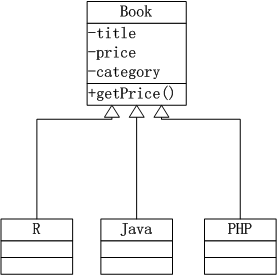``````
> Book <- R6Class("Book",            # 父类
+    private = list(
+      title=NA,
+      price=NA,
+      category=NA
+    ),
+   public = list(
+     initialize = function(title,price,category){
+       private\$title <- title
+       private\$price <- price
+       private\$category <- category
+     },
+     getPrice=function(){
+       private\$price
+     }
+   )
+ )

> R <- R6Class("R",     # 子类R图书
+    inherit = Book
+ )
> Java <- R6Class("JAVA",  # 子类JAVA图书
+   inherit = Book
+ )
> Php <- R6Class("PHP",    # 子类PHP图书
+   inherit = Book
+ )
``````

``````
> r1<-R\$new("R的极客理想-工具篇",59,"R")
> r1\$getPrice()
 59

> j1<-Java\$new("Java编程思想",108,"JAVA")
> j1\$getPrice()
 108

> p1<-Java\$new("Head First PHP & MySQL",98,"PHP")
> p1\$getPrice()
 98
``````

• 所有图书9折
• JAVA图书7折，不支持重复打折
• 为了推动R图书的销售，R语言图书7折，并支持重复打折
• PHP图书无特别优惠

``````
> Book <- R6Class("Book",
+   private = list(
+     title=NA,
+     price=NA,
+     category=NA
+   ),
+   public = list(
+     initialize = function(title,price,category){
+       private\$title <- title
+       private\$price <- price
+       private\$category <- category
+     },
+     getPrice=function(){
+       p<-private\$price*self\$discount()
+       print(paste("Price:",private\$price,", Sell out:",p,sep=""))
+     },
+     discount=function(){
+       0.9
+     }
+   )
+ )
``````

3个子类，分别对应自己的打折规则，分别进行修改。

• 给JAVA子类增加 discount()方法，用于覆盖父类的discount()方法，让JAVA图书7折，不支持重复打折，从而满足第二条规则。
• 给R子类增加 discount()方法，在子类的discount()方法中调用父类的discount()方法，让支持 R图书7折和9折的折上折，从而满足第三条规则。
• PHP子类，没有修改，完全遵循第一条规则的。
• ``````
> Java <- R6Class("JAVA",
+   inherit = Book,
+   public = list(
+     discount=function(){
+       0.7
+     }
+   )
+ )
>
> R <- R6Class("R",
+   inherit = Book,
+   public = list(
+     discount=function(){
+       super\$discount()*0.7
+     }
+   )
+ )
>
> Php <- R6Class("PHP",
+   inherit = Book
+ )
``````

分别查看3本图书的折后价格。

``````
> r1<-R\$new("R的极客理想-工具篇",59,"R")
> r1\$getPrice()
 "Price:59, Sell out:37.17"   # 59 * 0.9 *0.7= 37.17
>
> j1<-Java\$new("Java编程思想",108,"JAVA")
> j1\$getPrice()
 "Price:108, Sell out:75.6"    # 108 *0.7= 75.6
>
> p1<-Php\$new("Head First PHP & MySQL",98,"PHP")
> p1\$getPrice()
 "Price:98, Sell out:88.2"      # 98 *0.9= 88.2
``````

R图书打折最多，享受7折和9折的折上折优惠， 59 * 0.9 * 0.7= 37.17；Java图书享受7折优惠，108 *0.7= 75.6；PHP图书享受9折优惠 98 *0.9= 88.2。

通过这个实例，我们用R6的方法实现了面向对象编程中的封装、继承和多态的3个特征，证明R6是一种完全的面向对象的实现。R6类对象系统，提供了一种可兼容的面向对象实现方式，更接近于其他的编程语言上的面向对象的定义，由于R6底层基于S3来实现的，所以比RC的类更加有效果。

我们一共介绍了4种R语言的面向对象体系结构，选择自己理解的，总有一种会适合你。

转载请注明出处：
http://blog.fens.me/r-class-r6/## R语言基于RC的面向对象编程

R的极客理想系列文章，涵盖了R的思想，使用，工具，创新等的一系列要点，以我个人的学习和体验去诠释R的强大。

R语言作为统计学一门语言，一直在小众领域闪耀着光芒。直到大数据的爆发，R语言变成了一门炙手可热的数据分析的利器。随着越来越多的工程背景的人的加入，R语言的社区在迅速扩大成长。现在已不仅仅是统计领域，教育，银行，电商，互联网….都在使用R语言。

• 张丹(Conan), 程序员Java,R,PHP,Javascript
• weibo：@Conan_Z
• blog: http://blog.fens.me
• email: bsspirit@gmail.com

http://blog.fens.me/r-class-rc/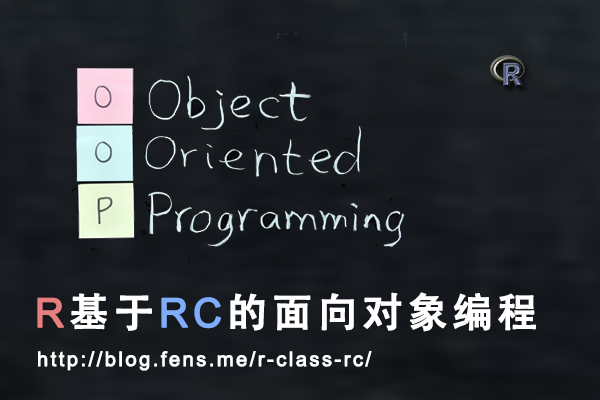RC对象系统从底层上改变了原有S3和S4对象系统的设计，去掉了泛型函数，真正地以类为基础实现面向对象的特征。

1. RC对象系统介绍
2. 创建RC类和对象
3. 对象赋值
4. 定义对象的方法
5. RC对象内置方法
6. RC类的辅助构造函数
7. RC对象系统的使用

## 1 RC对象系统介绍

RC是Reference classes的简称，又被称为R5，在R语言的2.12版本被引入的，是最新一代的面向对象系统。

RC不同于原来的S3和S4对象系统，RC对象系统的方法是在类中自定的，而不是泛型函数。RC对象的行为更相似于其他的编程语言，实例化对象的语法也有所改变。

• 类：面向对象系统的基本类型，类是静态结构定义。
• 对象：类实例化后，在内存中生成结构体。
• 方法：是类中的函数定义，不通过泛型函数实现。

## 2 创建RC类和对象

• Linux: Ubuntu Server 12.04.2 LTS 64bit
• R: 3.0.1 x86_64-pc-linux-gnu

``````
# 加载pryr包
> library(pryr)
``````

2.1 如何创建RC类？

RC对象系统是以类为基本类型， 有专门的类的定义函数 setRefClass() 和 实例化则通过类的方法生成，我们一下如何用RC对象系统创建一个类。

2.1.1 setRefClass()

``````
setRefClass(Class, fields = , contains = , methods =, where =, ...)
``````

• Class: 定义类名
• fields: 定义属性和属性类型
• contains: 定义父类，继承关系
• methods: 定义类中的方法
• where: 定义存储空间

2.2 创建RC类和实例

``````
# 定义一个RC类
> User<-setRefClass("User",fields=list(name="character"))

# 查看User的定义
> User
Generator for class "User":

Class fields:
Name:       name
Class: character

Class Methods:
"callSuper", "copy", "export", "field", "getClass",
"getRefClass", "import", "initFields", "show", "trace",
"untrace", "usingMethods"

Reference Superclasses:
"envRefClass"

# 实例化一个User对象u1
> u1<-User\$new(name="u1")

# 查看u1对象
> u1
Reference class object of class "User"
Field "name":
 "u1"

# 检查User类的类型
> class(User)
 "refObjectGenerator"
attr(,"package")
 "methods"
> is.object(User)
 TRUE
> otype(User)
 "RC"

# 检查u1的类型
> class(u1)
 "User"
attr(,"package")
 ".GlobalEnv"
> is.object(u1)
 TRUE
> otype(u1)
 "RC"
``````

2.3 创建一个有继承关系的RC类

``````
# 创建RC类User
> User<-setRefClass("User",fields=list(name="character"))

# 创建User的子类Member
> Member<-setRefClass("Member",contains="User",fields=list(manager="User"))

# 实例化User
> manager<-User\$new(name="manager")

# 实例化一个Son对象
> member<-Member\$new(name="member",manager=manager)

# 查看member对象
> member
Reference class object of class "Member"
Field "name":
 "member"
Field "manager":
Reference class object of class "User"
Field "name":
 "manager"

# 查看member对象的name属性
> member\$name
 "member

# 查看member对象的manager属性
> member\$manager
Reference class object of class "User"
Field "name":
 "manager"

# 检查对象的属性类型
> otype(member\$name)
 "primitive"
> otype(member\$manager)
 "RC"
``````

2.4 RC对象的默认值

RC的类有一个指定构造器方法 \$initialize()，这个构造器方法在实例化对象时，会自动被运行一次，通过这个构造方法可以设置属性的默认值。

``````
# 定义一个RC类
> User<-setRefClass("User",
+
+     # 定义2个属性
+     fields=list(name="character",level='numeric'),
+     methods=list(
+
+          # 构造方法
+          initialize = function(name,level){
+              print("User::initialize")
+
+              # 给属性增加默认值
+              name <<- 'conan'
+              level <<- 1
+           }
+      )
+ )

# 实例化对象u1
> u1<-User\$new()
 "User::initialize"

# 查看对象u1，属性被增加了默认值
> u1
Reference class object of class "User"
Field "name":
 "conan"
Field "level":
 1
``````

## 3 对象赋值

``````
# 定义User类
> User<-setRefClass("User",fields=list(name="character",age="numeric",gender="factor"))

# 定义一个factor类型
> genderFactor<-factor(c('F','M'))

# 实例化u1
> u1<-User\$new(name="u1",age=44,gender=genderFactor)

# 查看age属性值
> u1\$age
 44
``````

``````
# 重新赋值
> u1\$age<-10

# age属性改变
> u1\$age
 10
``````

``````
# 把u1赋值给u2对象
> u2<-u1

# 查看u2的age属性
> u2\$age
 10

# 重新赋值
> u1\$age<-20

# 查看u1,u2的age属性，都发生改变
> u1\$age
 20
> u2\$age
 20
``````

``````
# 调用u1的内置方法copy()，赋值给u3
> u3<-u1\$copy()

# 查看u3的age属性
> u3\$age
 20

# 重新赋值
> u1\$age<-30

# 查看u1的age属性，发生改变
> u1\$age
 30

# 查看u3的age属性，没有改变
> u3\$age
 20
``````

## 4 定义对象的方法

``````
# 定义一个RC类，包括方法
> User<-setRefClass("User",
+       fields=list(name="character",favorite="vector"),
+
+       # 方法属性
+       methods = list(
+
+           # 增加一个兴趣
+                 favorite <<- c(favorite,x)
+           },
+
+           # 删除一个兴趣
+           delFavorite = function(x) {
+                 favorite <<- favorite[-which(favorite == x)]
+           },
+
+           # 重新定义兴趣列表
+           setFavorite = function(x) {
+                 favorite <<- x
+           }
+       )
+ )

# 实例化对象u1
> u1<-User\$new(name="u1",favorite=c('movie','football'))

# 查看u1对象
> u1
Reference class object of class "User"
Field "name":
 "u1"
Field "favorite":
 "movie"    "football"
``````

``````
# 删除一个兴趣
> u1\$delFavorite('football')

# 查看兴趣属性
> u1\$favorite
 "movie"

# 增加一个兴趣
> u1\$favorite
 "movie"    "shopping"

# 重置兴趣列表
> u1\$favorite
``````

## 5 RC对象内置方法和内置属性

5.1 内置方法：

• initialize 类的初始化函数，用于设置属性的默认值，只能在类定义的方法中使用。
• callSuper 调用父类的同名方法，只能在类定义的方法中使用。
• copy 复制实例化对象的所有属性。
• initFields 给对象的属性赋值。
• field 查看属性或给属性赋值。
• getClass 查看对象的类定义。
• getRefClass 同getClass。
• show 查看当前对象。
• export 查看属性值以类为作用域。
• import 把一个对象的属性值赋值给另一个对象。
• trace 跟踪对象中方法调用，用于程序debug。
• untrace 取消跟踪。
• usingMethods 用于实现方法调用，只能在类定义的方法中使用。这个方法不利于程序的健壮性，所以不建议使用。

``````
# 类User
> User<-setRefClass("User",
+    fields=list(name="character",level='numeric'),
+    methods=list(
+      initialize = function(name,level){
+        print("User::initialize")
+        name <<- 'conan'
+        level <<- 1
+      },
+        level <<- level+x
+      },
+      }
+    )
+)
``````

``````
# 子类Member
> Member<-setRefClass("Member",contains="User",
+
+    # 子类中的属性
+    fields=list(age='numeric'),
+    methods=list(
+
+      # 覆盖父类的同名方法
+
+          # 调用父类的同名方法
+          callSuper(x)
+          level <<- level+1
+      }
+    )
+)
``````

``````
# 实例化u1
> u1<-User\$new(name='u1',level=10)
 "User::initialize"

# 查看u1对象，\$new()不能实现赋值的操作
> u1
Reference class object of class "User"
Field "name":
 "conan"
Field "level":
 1

# 通过\$initFields()向属性赋值
> u1\$initFields(name='u1',level=10)
Reference class object of class "User"
Field "name":
 "u1"
Field "level":
 10

# 实例化m1
> m1<-Member\$new()
 "User::initialize"

> m1\$initFields(name='m1',level=100,age=12)
Reference class object of class "Member"
Field "name":
 "m1"
Field "level":
 100
Field "age":
 12
``````

``````
# 属性复制到u2
> u2<-u1\$copy()
 "User::initialize"

Reference class object of class "User"
Field "name":
 "u1"
Field "level":
 11

# u2的level与u1没有引入关系，u2没有变化
> u2
Reference class object of class "User"
Field "name":
 "u1"
Field "level":
 10
``````

``````
# 查看level属性值
> u1\$field('level')
 11

# 给level赋值为1
> u1\$field('level',1)

# 查看level属性值
> u1\$level
 1
``````

``````
# 类引入的定义
> m1\$getRefClass()
Generator for class "Member":

Class fields:
Name:       name     level       age
Class: character   numeric   numeric

Class Methods:
"initialize", "show", "trace", "untrace", "usingMethods"

Reference Superclasses:
"User", "envRefClass"

# 类定义
> m1\$getClass()
Reference Class "Member":

Class fields:
Name:       name     level       age
Class: character   numeric   numeric

Class Methods:
"initialize", "show", "trace", "untrace", "usingMethods"

Reference Superclasses:
"User", "envRefClass"

# 通过otype查看类型的不同
> otype(m1\$getRefClass())
 "RC"
> otype(m1\$getClass())
 "S4"
``````

``````
> m1\$show()
Reference class object of class "Member"
Field "name":
 "m1"
Field "level":
 100
Field "age":
 12

> show(m1)
Reference class object of class "Member"
Field "name":
 "m1"
Field "level":
 100
Field "age":
 12

> m1
Reference class object of class "Member"
Field "name":
 "m1"
Field "level":
 100
Field "age":
 12
``````

``````
Tracing reference method "addLevel" for object from class "Member"

Untracing reference method "addLevel" for object from class "Member"
``````

``````
# 查看在Member类中的属性
> m1\$export('Member')
Reference class object of class "Member"
Field "name":
 "m1"
Field "level":
 105
Field "age":
 12

# 查看在User类中的属性，当前作用域不包括age属性。
> m1\$export('User')
 "User::initialize"
Reference class object of class "User"
Field "name":
 "m1"
Field "level":
 105
``````

``````
# 实例化m2
> m2<-Member\$new()
 "User::initialize"
> m2
Reference class object of class "Member"
Field "name":
 "conan"
Field "level":
 1
Field "age":
numeric(0)

# 把m1对象的值赋值给m2对象
> m2\$import(m1)
> m2
Reference class object of class "Member"
Field "name":
 "m1"
Field "level":
 105
Field "age":
 12
``````

5.2 内置属性：

RC对象实例化后，有两个内置属性：

• .self 实例化对象自身
• .refClassDef 类的定义类型
``````
# \$.self属性
> m1\$.self
Reference class object of class "Member"
Field "name":
 "m1"
Field "level":
 105
Field "age":
 12

#  m1\$.self和m1 完全相同
> identical(m1\$.self,m1)
 TRUE

# 查看类型
> otype(m1\$.self)
 "RC"

# \$.refClassDef属性
> m1\$.refClassDef
Reference Class "Member":

Class fields:
Name:       name     level       age
Class: character   numeric   numeric

Class Methods:
"initialize", "show", "trace", "untrace", "usingMethods"

Reference Superclasses:
"User", "envRefClass"

# 与\$getClass()相同
> identical(m1\$.refClassDef,m1\$getClass())
 TRUE

# 查看类型
> otype(m1\$.refClassDef)
 "S4"
``````

## 6 RC类的辅助函数

• new 用于实例化对象。
• help 用于查询类中方法的调用。
• methods 列出类中定义的所有方法。
• fields 列出类中定义的所有属性。
• lock 给属性加锁，实例化对象的属性只允许赋值一次，即赋值变量，不可修改。
• trace 跟踪方法。
• accessors 给属性生成get/set方法。

``````
# 定义User类
> User<-setRefClass("User",
+    fields=list(name="character",level='numeric'),
+    methods=list(
+      initialize = function(name,level){
+        print("User::initialize")
+        name <<- 'conan'
+        level <<- 1
+      },
+        level <<- level+x
+      },
+      }
+    )
+)

# 实例化对象u1
> u1<-User\$new()

# 列出User类中的属性
> User\$fields()
name       level
"character"   "numeric"

# 列出User类中的方法
> User\$methods()
 "copy"         "export"       "field"
 "getClass"     "getRefClass"  "import"
 "initFields"   "initialize"   "show"
 "trace"        "untrace"      "usingMethods"

# 查看User类的方法调用
Call:

> User\$help("show")
Call:
\$show()
``````

``````
# 给level属性增加get/set方法
> User\$accessors("level")

# 给name属性增加get/set方法
> User\$accessors("name")

# 列出所有方法
> User\$methods()
 "copy"         "export"       "field"
 "getClass"     "getLevel"     "getName"
 "getRefClass"  "import"       "initFields"
 "initialize"   "setLevel"     "setName"
 "show"         "trace"        "untrace"
 "usingMethods"
``````

``````
Tracing reference method "addLevel" for class
"User"

# 实例化对象u3
> u3<-User\$new(name='u3',level=1)
 "User::initialize"

``````

``````
# 锁定level属性
> User\$lock("level")

# 查看User类中被锁定的属性
> User\$lock()
 "level"

# 实例化对象u3，这时level属性已经被赋值一次
> u3<-User\$new()
 "User::initialize"
> u3
Reference class object of class "User"
Field "name":
 "conan"
Field "level":
 1

# 给level属性，再次赋值出错
> u3\$level=1
Error: invalid replacement: reference class field ‘level’ is read-only
Error: invalid replacement: reference class field ‘level’ is read-only
``````

## 7 RC对象系统的使用

7.1 任务一：定义动物的数据结构和发声方法。

• 定义动物的数据结构
• 分别定义3种动物的发声bark()方法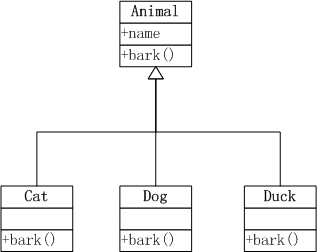``````
# 创建Animal类，包括name属性，构造方法initialize()，叫声方法bark()。
> Animal<-setRefClass("Animal",
+  fields=list(name="character"),
+  methods=list(
+    initialize = function(name) name <<- 'Animal',
+    bark = function() print("Animal::bark")
+  )
+)

# 创建Cat类，继承Animal类，并重写(Overwrite)了 initialize() 和 bark()。
> Cat<-setRefClass("Cat",contains="Animal",
+  methods=list(
+    initialize = function(name) name <<- 'cat',
+    bark = function() print(paste(name,"is miao miao"))
+  )
+)

# 创建Dog类，继承Animal类，并重写(Overwrite)了 initialize() 和 bark()。
> Dog<-setRefClass("Dog",contains="Animal",
+  methods=list(
+    initialize = function(name) name <<- 'dog',
+    bark = function() print(paste(name,"is wang wang"))
+  )
+)

# 创建Duck类，继承Animal类，并重写(Overwrite)了 initialize() 和 bark()。
> Duck<-setRefClass("Duck",contains="Animal",
+   methods=list(
+     initialize = function(name) name <<- 'duck',
+     bark = function() print(paste(name,"is ga ga"))
+   )
+)
``````

``````
# 创建cat实例
> cat<-Cat\$new()
> cat\$name
 "cat"

# cat叫声
> cat\$bark()
 "cat is miao miao"

# 创建dog实例，并给dog起名叫Huang
> dog<-Dog\$new()
> dog\$initFields(name='Huang')
Reference class object of class "Dog"
Field "name":
 "Huang"
> dog\$name
 "Huang"

# dog叫声
> dog\$bark()
 "Huang is wang wang"

# 创建duck实例
> duck<-Duck\$new()

# duck叫声
> duck\$bark()
 "duck is ga ga"
``````

7.2 任务二：定义动物的体貌特征

3种动物都有肢，cat和dog是四肢，duck是二肢和二翅。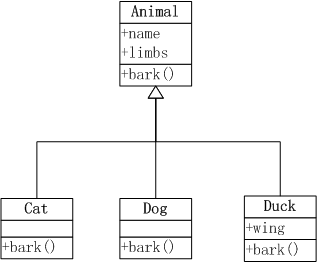``````
# 定义Animal类，增加limbs属性，默认值为4
Animal<-setRefClass("Animal",
fields=list(name="character",limbs='numeric'),
methods=list(
initialize = function(name) {
name <<- 'Animal'
limbs<<-4
},
bark = function() print("Animal::bark")
)
)

# 在Cat类的 initialize()方法中，执行callSuper()方法，调用父类的同名方法
Cat<-setRefClass("Cat",contains="Animal",
methods=list(
initialize = function(name) {
callSuper()
name <<- 'cat'
},
bark = function() print(paste(name,"is miao miao"))
)
)

# 在Dog类的 initialize()方法中，执行callSuper()方法，调用父类的同名方法
Dog<-setRefClass("Dog",contains="Animal",
methods=list(
initialize = function(name) {
callSuper()
name <<- 'dog'
},
bark = function() print(paste(name,"is wang wang"))
)
)

# 在Dog类的定义wing属性， 并在initialize()方法，定义limbs和wing属性的默认值
Duck<-setRefClass("Duck",contains="Animal",
fields=list(wing='numeric'),
methods=list(
initialize = function(name) {
name <<- 'duck'
limbs<<- 2
wing<<- 2
},
bark = function() print(paste(name,"is ga ga"))
)
)
``````

``````
# 实例化cat对象，属性limbs为4
> cat<-Cat\$new();cat
Reference class object of class "Cat"
Field "name":
 "cat"
Field "limbs":
 4

# 实例化dog对象，属性limbs为4
> dog<-Dog\$new()
> dog\$initFields(name='Huang')
Reference class object of class "Dog"
Field "name":
 "Huang"
Field "limbs":
 4
> dog
Reference class object of class "Dog"
Field "name":
 "Huang"
Field "limbs":
 4

# 实例化duck对象，属性limbs为2，wing为2
> duck<-Duck\$new();duck
Reference class object of class "Duck"
Field "name":
 "duck"
Field "limbs":
 2
Field "wing":
 2
``````

7.3 任务三：定义动物的行动方式。

• 猫(cat) 爬树
• 狗(dog) 游泳
• 鸭(duck) 游泳，短距离飞行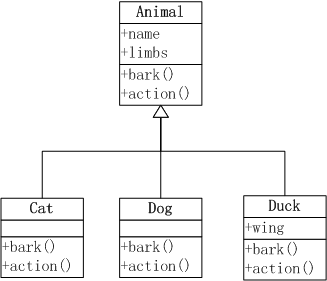``````
# 定义类Animal，增加action()方法，用于通用的行为陆地上行动。
> Animal<-setRefClass("Animal",
+    fields=list(name="character",limbs='numeric'),
+    methods=list(
+      initialize = function(name) {
+        name <<- 'Animal'
+        limbs<<-4
+      },
+      bark = function() print("Animal::bark"),
+      action = function() print("I can walk on the foot")
+    )
+)

# 定义Cat类，重写action()方法，并增加爬树的行动
> Cat<-setRefClass("Cat",contains="Animal",
+     methods=list(
+       initialize = function(name) {
+         callSuper()
+         name <<- 'cat'
+       },
+       bark = function() print(paste(name,"is miao miao")),
+       action = function() {
+         callSuper()
+         print("I can Climb a tree")
+       }
+     )
+  )

# 定义Dog类，重写action()方法，并增加游泳行动
> Dog<-setRefClass("Dog",contains="Animal",
+   methods=list(
+     initialize = function(name) {
+       callSuper()
+       name <<- 'dog'
+     },
+     bark = function() print(paste(name,"is wang wang")),
+     action = function() {
+         callSuper()
+         print("I can Swim.")
+     }
+   )
+)

# 定义Duck类，重写action()方法，并增加游泳和短距离飞行
> Duck<-setRefClass("Duck",contains="Animal",
+    fields=list(wing='numeric'),
+    methods=list(
+      initialize = function(name) {
+        name <<- 'duck'
+        limbs<<- 2
+        wing<<- 2
+      },
+      bark = function() print(paste(name,"is ga ga")),
+      action = function() {
+        callSuper()
+        print("I can swim.")
+        print("I also can fly a short way.")
+     }
+    )
+)
``````

cat的行动。

``````
# 实例化cat
> cat<-Cat\$new()

# cat的行动
> cat\$action()
 "I can walk on the foot"
 "I can Climb a tree"
``````

dog的行动。

``````
> dog<-Dog\$new()
> dog\$action()
 "I can walk on the foot"
 "I can Swim."
``````

duck的行动。

``````
> duck<-Duck\$new()
> duck\$action()
 "I can walk on the foot"
 "I can swim."
 "I also can fly a short way."
``````

http://blog.fens.me/r-class-rc/## R语言基于S3的面向对象编程

R的极客理想系列文章，涵盖了R的思想，使用，工具，创新等的一系列要点，以我个人的学习和体验去诠释R的强大。

R语言作为统计学一门语言，一直在小众领域闪耀着光芒。直到大数据的爆发，R语言变成了一门炙手可热的数据分析的利器。随着越来越多的工程背景的人的加入，R语言的社区在迅速扩大成长。现在已不仅仅是统计领域，教育，银行，电商，互联网….都在使用R语言。

• 张丹(Conan), 程序员Java,R,PHP,Javascript
• weibo：@Conan_Z
• blog: http://blog.fens.me
• email: bsspirit@gmail.com

http://blog.fens.me/r-class-s3/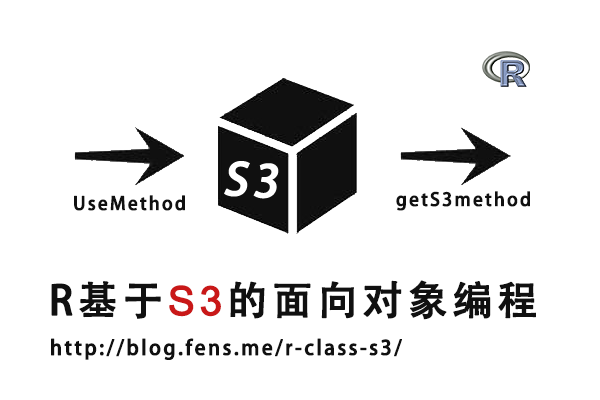S3对象简单、具有动态性、结构化特征不明显；S4对象结构化、 功能强大；RC对象是2.12版本后使用的新类型，用于解决S3,S4很难实现的对象。

1. S3对象介绍
2. 创建S3对象
3. 泛型函数和方法调用
4. 查看S3对象的函数
5. S3对象继承
6. S3对象的缺点
7. S3对象的使用

## 2 创建S3对象

• Linux: Ubuntu Server 12.04.2 LTS 64bit
• R: 3.0.1 x86_64-pc-linux-gnu

``````
# 加载pryr包
> library(pryr)
``````

``````
> x<-1
> attr(x,'class')<-'foo'

> x
 1
attr(,"class")
 "foo"

> class(x)
 "foo"

# 用pryr包的otype函数，检查x的类型
> otype(x)
 "S3"
``````

``````
> y <- structure(2, class = "foo")

> y
 2
attr(,"class")
 "foo"

> class(y)
 "foo"

> otype(y)
 "S3"
``````

S3对象没有明确结构关系，一个S3对象可以有多个类型, S3对象的 class 属性可以是一个向量，包括多种类型。

``````
> x<-1
> attr(x,'class')<- c("foo", "bar")
> class(x)
 "foo" "bar"
> otype(x)
 "S3"
``````

## 3 泛型函数和方法调用

+ 用UseMethod()定义teacher泛型函数
+ 用teacher.xxx的语法格式定义teacher对象的行为
+ 其中teacher.default是默认行为

``````
# 用UseMethod()定义teacher泛型函数
> teacher <- function(x, ...) UseMethod("teacher")

# 用pryr包中ftype()函数，检查teacher的类型
> ftype(teacher)
 "s3"      "generic"

# 定义teacher内部函数
> teacher.lecture <- function(x) print("讲课")
> teacher.assignment <- function(x) print("布置作业")
> teacher.correcting <- function(x) print("批改作业")
> teacher.default<-function(x) print("你不是teacher")
``````

• 定义一个变量a，并设置a的class属性为lecture
• 把变量a，传入到teacher泛型函数中
• 函数teacher.lecture()函数的行为被调用
``````
> a<-'teacher'

# 给老师变量设置行为
> attr(a,'class') <- 'lecture'

# 执行老师的行为
> teacher(a)
 "讲课"
``````

``````
> teacher.lecture()
 "讲课"

> teacher.lecture(a)
 "讲课"

> teacher()
 "你不是teacher"
``````

## 4 查看S3对象的函数

``````
# 查看teacher对象
> teacher
function(x, ...) UseMethod("teacher")

# 查看teacher对象的内部函数
>  methods(teacher)
 teacher.assignment teacher.correcting teacher.default    teacher.lecture
``````

``````
> methods(generic.function=predict)
 predict.ar*                predict.Arima*             predict.arima0*
 predict.glm                predict.HoltWinters*       predict.lm
 predict.loess*             predict.mlm                predict.nls*
 predict.poly               predict.ppr*               predict.prcomp*
 predict.princomp*          predict.smooth.spline*     predict.smooth.spline.fit*
 predict.StructTS*

Non-visible functions are asterisked
``````

``````
> methods(class=lm)
 confint.lm*        cooks.distance.lm* deviance.lm*       dfbeta.lm*
 dfbetas.lm*        drop1.lm*          dummy.coef.lm*     effects.lm*
 extractAIC.lm*     family.lm*         formula.lm*        hatvalues.lm
 influence.lm*      kappa.lm           labels.lm*         logLik.lm*
 model.frame.lm     model.matrix.lm    nobs.lm*           plot.lm
 predict.lm         print.lm           proj.lm*           qr.lm*
 residuals.lm       rstandard.lm       rstudent.lm        simulate.lm*
 summary.lm         variable.names.lm* vcov.lm*

Non-visible functions are asterisked
``````

``````
# 查看teacher.lecture函数
> getAnywhere(teacher.lecture)
A single object matching ‘teacher.lecture’ was found
It was found in the following places
.GlobalEnv
registered S3 method for teacher
with value

function(x) print("讲课")

# 查看不可见的函数predict.ppr
> predict.ppr
> exists("predict.ppr")
 FALSE

# getAnywhere()函数查找predict.ppr
> getAnywhere("predict.ppr")
A single object matching ‘predict.ppr’ was found
It was found in the following places
registered S3 method for predict from namespace stats
namespace:stats
with value

function (object, newdata, ...)
{
if (missing(newdata))
return(fitted(object))
if (!is.null(object\$terms)) {
newdata <- as.data.frame(newdata)
rn <- row.names(newdata)
Terms <- delete.response(object\$terms)
m <- model.frame(Terms, newdata, na.action = na.omit,
xlev = object\$xlevels)
if (!is.null(cl <- attr(Terms, "dataClasses")))
.checkMFClasses(cl, m)
keep <- match(row.names(m), rn)
x <- model.matrix(Terms, m, contrasts.arg = object\$contrasts)
}
else {
x <- as.matrix(newdata)
keep <- seq_len(nrow(x))
rn <- dimnames(x)[[1L]]
}
if (ncol(x) != object\$p)
stop("wrong number of columns in 'x'")
res <- matrix(NA, length(keep), object\$q, dimnames = list(rn,
object\$ynames))
res[keep, ] <- matrix(.Fortran(C_pppred, as.integer(nrow(x)),
as.double(x), as.double(object\$smod), y = double(nrow(x) *
object\$q), double(2 * object\$smod[4L]))\$y, ncol = object\$q)
drop(res)
}
<bytecode: 0x000000000df6c2d0>
<environment: namespace:stats>
``````

``````
# getS3method()函数查找predict.ppr
> getS3method("predict", "ppr")
function (object, newdata, ...)
{
if (missing(newdata))
return(fitted(object))
if (!is.null(object\$terms)) {
newdata <- as.data.frame(newdata)
rn <- row.names(newdata)
Terms <- delete.response(object\$terms)
m <- model.frame(Terms, newdata, na.action = na.omit,
xlev = object\$xlevels)
if (!is.null(cl <- attr(Terms, "dataClasses")))
.checkMFClasses(cl, m)
keep <- match(row.names(m), rn)
x <- model.matrix(Terms, m, contrasts.arg = object\$contrasts)
}
else {
x <- as.matrix(newdata)
keep <- seq_len(nrow(x))
rn <- dimnames(x)[[1L]]
}
if (ncol(x) != object\$p)
stop("wrong number of columns in 'x'")
res <- matrix(NA, length(keep), object\$q, dimnames = list(rn,
object\$ynames))
res[keep, ] <- matrix(.Fortran(C_pppred, as.integer(nrow(x)),
as.double(x), as.double(object\$smod), y = double(nrow(x) *
object\$q), double(2 * object\$smod[4L]))\$y, ncol = object\$q)
drop(res)
}
<bytecode: 0x000000000df6c2d0>
<environment: namespace:stats>
``````

## 5 S3对象的继承关系

S3对象有一种非常简单的继承方式，用NextMethod()函数来实现。

``````
> node <- function(x) UseMethod("node", x)
> node.default <- function(x) "Default node"

# father函数
> node.father <- function(x) c("father")

# son函数，通过NextMethod()函数指向father函数
> node.son <- function(x) c("son", NextMethod())

# 定义n1
> n1 <- structure(1, class = c("father"))
# 在node函数中传入n1，执行node.father()函数
> node(n1)
 "father"

# 定义n2，设置class属性为两个
> n2 <- structure(1, class = c("son", "father"))
# 在node函数中传入n2，执行node.son()函数和node.father()函数
> node(n2)
 "son"    "father"
``````

## 6 S3对象的缺点

• S3使用起来简单，但在实际的面向对象编程过程中，当对象关系有一定的复杂度，S3对象所表达的意义就会变得不太清楚。
• S3封装的内部函数，可绕过泛型函数的检查，以直接被调用。
• S3参数的class属性，可以被任意设置，没有预处理的检查。
• S3参数，只能通过调用class属性进行函数调用，其他属性则不会被class()函数执行。
• S3参数的class属性有多个值时，调用时会按照程序赋值顺序来调用第一个合法的函数。

## 7 S3对象的使用

S3对象系统，被广泛地应用于R语言的早期开发中。在base包中，就有很多的S3对象。

base包的S3对象

``````
# mean函数
> mean
function (x, ...)
UseMethod("mean")

> ftype(mean)
 "s3"      "generic"

# t函数
> ftype(t)
 "s3"      "generic"

# plot函数
> ftype(plot)
 "s3"      "generic"
``````

``````
# 定义数字型变量a
> a <- 1
# 变量a的class为numeric
> class(a)
 "numeric"

# 定义泛型函数f1
> f1 <- function(x) {
+   a <- 2
+   UseMethod("f1")
+ }

# 定义f1的内部函数
> f1.numeric <- function(x) a

# 给f1()传入变量a
> f1(a)
 2

# 给f1()传入数字99
> f1(99)
 2

# 定义f1的内部函数
> f1.character <- function(x) paste("char", x)

# 给f1()传入字符a
> f1("a")
 "char a"
``````

http://blog.fens.me/r-class-s3/